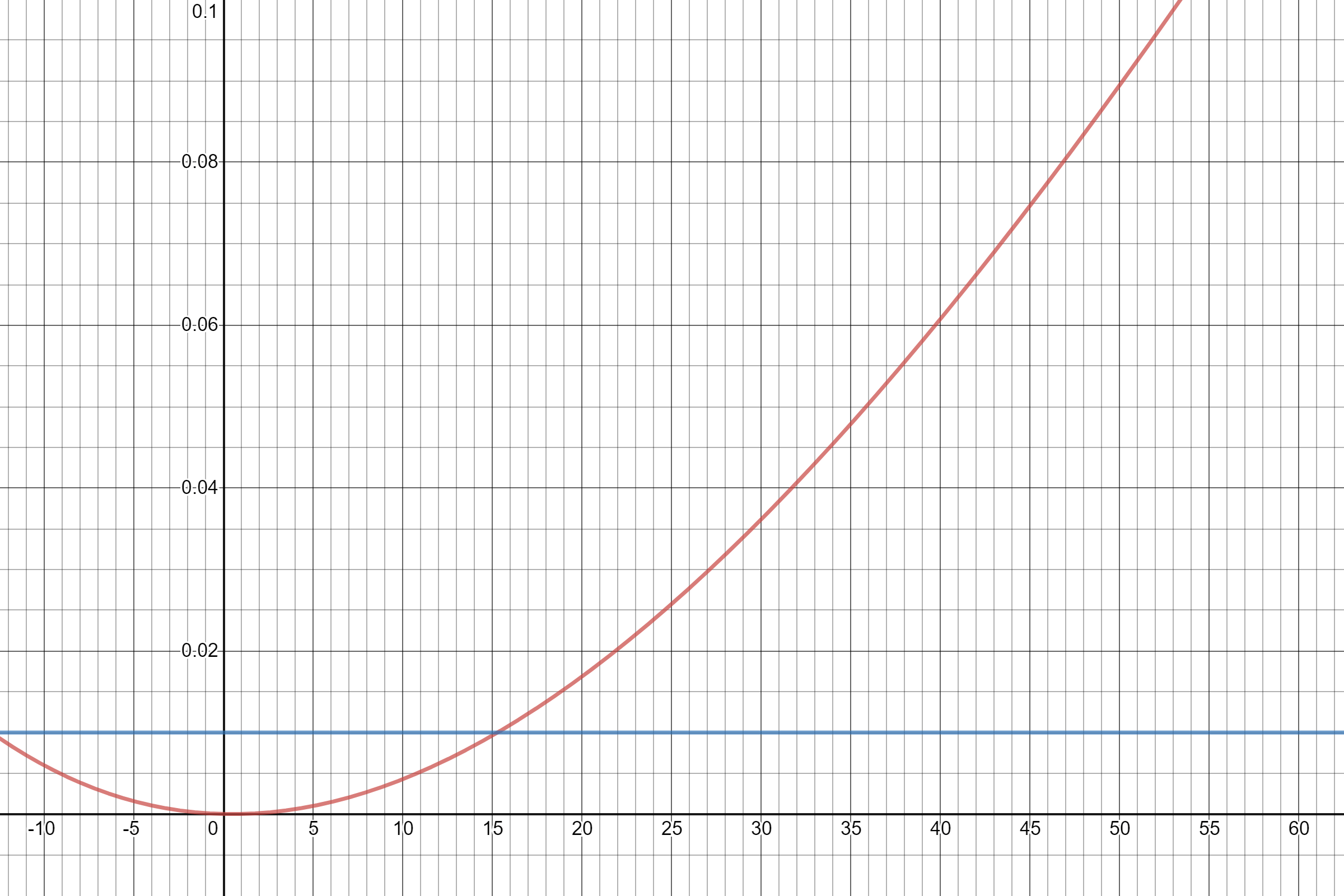Browse Month

# Limitations of the Bus FactorOver lunch today, a friend and I discussed what we were doing in response to COVID-19. I mentioned I was working from home, and that the purpose of working from home was presumably to avoid the offices becoming a coronavirus cluster. This isn’t the same as quarantine or self-isolation in that I can and do still leave the house, attend smaller-scale social events and meet friends. The probability seems not too high, but even if I become sick the idea is that we don’t want this to spread to others on the team or at the company.

The bus factor is a simple measurement of how concentrated key personnel are in a team. It is defined as the minimum number of people that can be removed from a team before the project stalls. For example, if a shop has five employees, where everyone can restock shelves but only two people are authorised to operate the cash register, then the shop team has a bus factor of 2, since if those two people are removed the shop will not function.

Generally, a higher number is better, as it means the team can tolerate more people being unavailable. This is often used to inform risk planning, such as by prioritising context-sharing, developer documentation or other means of spreading knowledge.

However, there is quite a lot of variance within a single measurement. For example, suppose we have a team A which has two leads, and we say that the team will stall if both of them are unavailable (but only them – any other group of people becoming unavailable, including everyone but one of the leads, is okay). Also consider team B, where the absence of any two people will cause the team to stall (though no individual would). Both teams have a bus factor of 2. However, A is strictly safer than B, and it seems to be quite a large margin. I’m not sure that B is safer than a team C, where there is one absolutely critical lead (bus factor 1), for that matter.

This could get us to a concrete problem. Suppose there is a 1 percent probability that each person on a team becomes unavailable for some reason. What is the smallest number of people such that it is possible that a team with a bus factor of 2 is actually riskier than a team with a bus factor of 1?

#### Finding the Extremes

To answer this question, we want to find the extremes of a given Bus Factor$BF$ in terms of risk, because we want to compare the riskiest Bus Factor 2 team against the safest Bus Factor 1 team. This drops out quite naturally: the safest team is the one where there exists one specific set of$BF$ people which will cause operations to stall, and no other combination that is not a superset of that set is essential. Conversely, the riskiest team is one where every combination of$BF$ people is critical. As defined above, team B is the riskiest possible Bus Factor 2 team, and team C is the safest possible Bus Factor 1 team.

Without loss of generality, let the people in the team be numbered$1, 2, \ldots, n$ and the lead have number 1 (or 1 and 2 in the case of team A). Let the probability that an entity$E$ becomes unavailable be$P(E_\times)$. Then, the probability that each team’s work stalls is as follows:$\displaystyle P(A_\times) = P(1_\times \wedge 2_\times)$$\displaystyle P(B_\times) = P \left( \bigvee_{i=1}^{n} \bigvee_{j=i+1}^{n} i_\times \wedge j_\times \right)$$\displaystyle P(C_\times) = P(1_\times)$

These terms as written aren’t very comparable – depending on the underlying probability distributions.

#### Attempting to Compare Terms

I’ve used logical formulas above to avoid making claims about the underlying probability distributions. However, if we assume that the probabilities are independently and identically distributed, and we say that over some fixed period of time the probability that an individual becomes unavailable is$p$, then we have$\displaystyle P(A_\times) = p^2$$\displaystyle P(C_\times) = p$$P(B_\times)$ is marginally trickier. This is the probability there are at least two people who become unavailable. It is probably easier to work out the complement$P(B_\times')$: that is the probability zero people or one person is unavailable. If we define$X$ as the number of people (out of$n$) who become unavailable, then$P(B_\times') = P(X=0) + P(X=1)$. It’s clear that$P(X=0) = (1-p)^n$.$P(X=1) = np(1-p)^{n-1}$: the probability a specific person is unavailable and no others are is$p(1-p)^{n-1}$, but there are$n$ valid versions of this. More generally,$X$ follows what’s called a binomial distribution. Thus,$\displaystyle P(B_\times') = (1-p)^n + np(1-p)^{n-1} = (1-p)^{n-1} \left( 1 - p + np \right)$

and thus$P(B_\times) = 1 - (1-p)^{n-1} \left( 1 - p + np \right)$

Notice that, as expected, this is dependent on$n$, while the other teams$A$ and$C$ have probabilities that don’t depend on$n$. Furthermore, as$n$ increases,$P(B_\times)$ increases – intuitively, as you consider more people, every way in which the team was already broken ignoring the new people remains broken, but some previously unbroken states can also break. (A more mathematical argument could look at the ratios of successive terms.)

#### Synthesis

The combination of exponential and polynomial (well, linear) terms in$P(B_\times)$ would make direct comparison with$P(C_\times)$ difficult. We can however fix some risk probability$p$ and find out at which value of$n$ team B (no one is individually critical, but every pair of people is critical) has a greater probability of stalling than team C (there is a single critical lead). To answer our initial question, we choose$p=0.01$. Since we have a closed form for the probability of team B having issues, we can plot a graph:It turns out the answer here,$n=16$ is actually relatively small in computational terms, even if it is large as far as teams are concerned. That said, the conditions for a team being the safest possible for a given bus factor are extremely strict – the sheer under-resourcing from removing people, even if they aren’t information silos or have unique knowledge, would still severely impair team function.